# Keeping Track of Element Order in Multiphysics Models

February 22, 2016

Whenever you are building a finite element model in COMSOL Multiphysics, you should be aware of the element order that is being used. This is particularly important for multiphysics models as there are some distinct benefits to using different element orders for different physics. Today, we will review the key concepts behind element order and discuss how it applies to some common multiphysics models.

### What Is Element Order?

Whenever we solve a finite element problem, we are approximating the true solution field to a partial differential equation (PDE) over a domain. The finite element method starts by subdividing the modeling domain up into smaller, simpler domains called elements. These elements are defined by a set of points, traditionally called nodes, and each node has a set of shape functions or basis functions. Every shape function is associated with some degrees of freedom. The set of all of these discrete degrees of freedom is traditionally referred to as the solution vector.

Note: You can read more about the process of going from the governing PDE to the solution vector in our previous blog posts “A Brief Introduction to the Weak Form” and “Discretizing the Weak Form Equations“.

Once the solution vector is computed, the finite element approximation to the solution field is constructed by interpolation using the solution vector and the set of all of the basis functions in all of the elements. The element order refers to the type of basis functions that are used.

Let’s now visualize some of the basis functions for one of the more commonly used elements in COMSOL Multiphysics: the two-dimensional Lagrange element. We will look at a square domain meshed with a single quadrilateral (four-sided) element that has a node at each corner. If we are computing a scalar field, then the Lagrange element has a single degree of freedom at each node. You can visualize the shape functions for a first-order Lagrange element in the image below.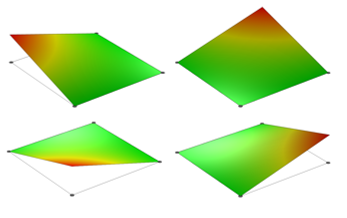The shape functions for a first-order square quadrilateral Lagrange element.

The first-order shape functions are each unity at one node and zero at all of the others. The complete finite element solution over this element is the sum of each shape function times its associated degree of freedom. We’ll now compare our first-order shape functions with our second-order shape functions.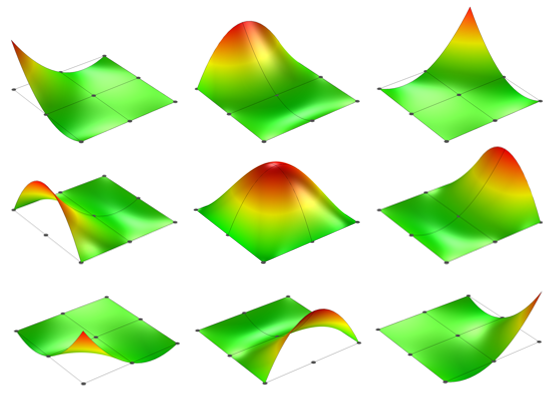The shape functions for a single second-order square quadrilateral Lagrange element.

Observe that the second-order quadrilateral Lagrange element has node points at the midpoints of the sides as well as in the element center. It has a total of nine shape functions and, again, each shape function is unity at one node and zero elsewhere.

Let’s now look at what happens when our single quadrilateral element represents a domain that is not a perfect square but rather a domain with some curved sides. In such cases, it is common to use a so-called isoparametric element, meaning that the geometry is approximated with the same shape functions as the one used for the solution. This geometric approximation is shown below for the first- and second-order cases.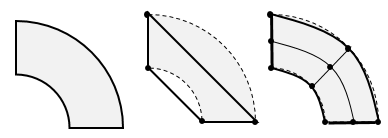A domain with curved sides. Single first- and second-order quadrilateral elements are applied.

As we can see in the image above, the first-order element simply approximates the curved sides as straight sides. The second-order element much more accurately approximates these curved boundaries. This difference, known as a geometric discretization error, is discussed in greater detail in an earlier blog post. The shape functions for the isoparametric first- and second-order Lagrange elements are shown below.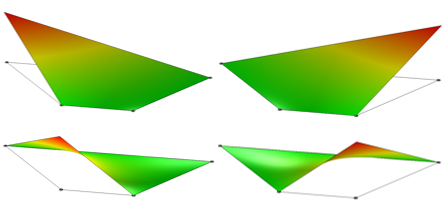The shape functions of a single first-order isoparametric Lagrange element for the domain with curved sides.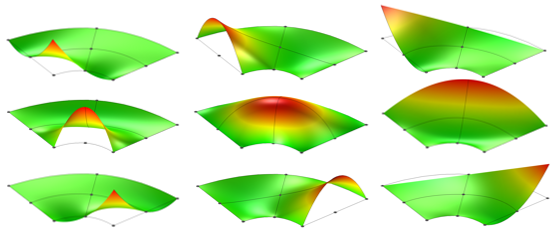The shape functions of a single second-order isoparametric Lagrange element for a domain with curved sides.

We can observe from the above two images that the first-order element approximates all sides of the domain as straight lines, while the second-order element approximates the curved shapes much more accurately. Thus, if we are modeling a domain with curved sides, we need to use several linear elements along any curved domain boundaries just so that we can accurately represent the domain itself.

For any real-world finite element model, there will of course always be more than one element describing the geometry. Additionally, keep in mind that regardless of the element order, you will want to perform a mesh refinement study, also called a mesh convergence study. That is, you will use finer and finer meshes (smaller and smaller elements) to solve the same problem and see how the solution converges. You terminate this mesh refinement procedure after achieving your desired accuracy. A good example of a mesh refinement study is presented in the application example of a Stress Analysis of an Elliptic Membrane.

All well-posed, single-physics finite element problems will converge toward the same answer, regardless of the element order. However, different element orders will converge at different rates and therefore require various computational resources. Let’s explore why different PDEs have different element orders.

### Element Order in Single-Physics Models

For the purposes of this discussion, let’s consider just the set of PDEs governing common single-physics problems that exhibit no variation in time. We can put all of these PDEs into one of two broad categories:

1. Poisson-type: Poisson-type PDEs are used to describe heat transfer in solids, solid mechanics, electric currents, electrostatics and magnetostatics, thin-film flow, and flow in porous media governed by Darcy’s law or the Richards’ equation. Such governing PDEs are all of the form:
\nabla \cdot (- D \nabla u ) = f

Note that this is a second-order PDE, thus second-order (quadratic) elements are the default choice within COMSOL Multiphysics for all of these types of equations.

2. Transport-type: Transport-type PDEs are used to describe chemical species transport as well as heat transfer in fluids and porous media. The governing equations here are quite similar to Poisson’s equation, with one extra term — a velocity vector:
\nabla \cdot ( -D \nabla u + \mathbf{v} u ) = f

The extra velocity term results in a governing equation that is closer to a first-order PDE. The velocity field is usually computed by solving the Navier-Stokes equation, which is itself a type of transport equation that describes fluid flow. It is often the case that, for such problems, there is a high Péclet number or Reynolds number. This is one of the reasons why the default choice is to use first-order (linear) elements for these PDEs.

Note that for fluid flow problems where the Reynolds number is low, the default is to use the so-called P2 + P1 elements that solve for the fluid velocity via second-order discretization and solve for the pressure via first-order discretization. The P2 + P1 elements are the default for the Creeping Flow, Brinkman Equations and Free and Porous Media Flow interfaces. This is also the case for the Two-Phase Flow, Level Set and Two-Phase Flow, Phase Field interfaces. Further, any type of transport or fluid flow interface uses stabilization to solve the problem more quickly and robustly. For an overview of stabilization methods, check out our earlier blog post “Understanding Stabilization Methods“.

So how can we check the default settings for the element order used by a particular physics interface? Within the Model Builder, we first need to go to the Show menu and toggle on Discretization. After doing so, you will see a Discretization section within the physics interface settings, as shown in the screenshot below.Screenshot showing how to view the element order of a physics interface.

Keep in mind that as long as you’re working with only single physics, it typically does not matter too much which element order you use as long as you remember to perform a mesh convergence study. The solutions with a different element order may require quite varying amounts of memory and time to solve, but they will all converge toward the same solution with sufficient mesh refinement. However, when we start dealing with multiphysics problems, things become a little bit more complicated. Next, we’ll look at two special cases of multiphysics modeling where you should be aware of element order.

### Conjugate Heat Transfer: Heat Transfer in Solids with Heat Transfer in Fluids

COMSOL Multiphysics includes a predefined multiphysics coupling between heat transfer and fluid flow that is meant for simulating the temperature of objects that are cooled or heated by a surrounding fluid. The Conjugate Heat Transfer interface (and the functionally equivalent Non-Isothermal Flow interface) is available with the Heat Transfer Module and the CFD Module for both laminar and turbulent fluid flow.

The Conjugate Heat Transfer interface is composed of two physics interfaces: the Heat Transfer interface and the Fluid Flow interface. The Fluid Flow interface (whether laminar or turbulent) uses linear element order to solve for the fluid velocity and pressure fields. The Heat Transfer interface solves for the temperature field in the fluid as well as the temperature field in the solid. The same linear element discretization is used throughout the temperature field in both the solid and fluid domains.

Now, if you are setting up a conjugate heat transfer problem by manually adding the various physics interfaces, you do need to be careful. If you start with the Heat Transfer in Solids interface and add a Heat Transfer in Fluids domain feature to the interface, a second-order discretization will be used for the temperature field by default. This is not generally advised, as it will require more memory than a first-order temperature discretization. The default first-order discretization of the fluid flow field justifies using first-order elements throughout the model.

It is also worth mentioning a related multiphysics coupling: the Local Thermal Non-Equilibrium interface available with the Heat Transfer Module. This interface is designed to solve for the temperature field of a fluid flowing through a porous matrix medium as well as the temperature of the matrix through which the fluid flows. That is, there are two different temperatures, the fluid and the solid matrix temperature, at each point in space. The interface also uses first-order discretization for both of the temperatures.

### Thermal Stress: Heat Transfer in Solids with Solid Mechanics

The other common case where a multiphysics coupling uses different element orders from a single-physics problem is when computing thermal stresses. For the Thermal Stress multiphysics coupling, the default is to use linear discretization for the temperature and quadratic discretization for the structural displacements. To understand why this is so, we can look at the governing Poisson-type PDE for linear elasticity:

\nabla \cdot ( \mathbf{C : \epsilon} ) = 0

where \mathbf{C} is the stiffness tensor and \mathbf{\epsilon} is the strain tensor.

For a problem where temperature variation affects stresses, the strain tensor is:

\mathbf{\epsilon} = \frac{1}{2}\left[ (\nabla \mathbf{u})^T + \nabla \mathbf{u}\right]-\alpha(T-T_0)

where \mathbf{\alpha} is a tensor containing the coefficients of thermal expansion, T is the temperature, T_0 is the strain-free reference temperature, and \mathbf{u} is the structural displacement field.

By default, we solve for the structural displacements using quadratic discretization, but we can see from the equation above that the strains are computed by taking the gradients of the displacement fields. This lowers the discretization order of the strains to a linear order. Hence, the temperature field discretization should also be lowered to a linear order.

### Closing Remarks

We have discussed the meaning of discretization order in COMSOL Multiphysics and why it is relevant for two different multiphysics cases that frequently arise. If you are putting together your own multiphysics models, you’ll want to keep element order in mind.

Additionally, it is good to address what can happen if you build a multiphysics model with element orders that disagree with what we’ve outlined here. As it turns out, in many cases, the worst thing that will happen is that your model will simply require more memory and converge to a solution more slowly. In the limit of mesh refinement, any combination of element orders in different physics will give the same results, but the convergence may well be very slow and oscillatory. If you do observe any spatial oscillations to the solution (for example, a stress field that looks rippled or wavy), then check the element orders.

Today’s blog post is designed as a practical guideline for element selection in multiphysics problems within COMSOL Multiphysics. A more in-depth discussion of stability criterion for mixed (hybrid) finite element methods can be found in many texts, such as Concepts and Applications of Finite Element Analysis by Robert D. Cook, David S. Malkus, Michael E. Plesha, and Robert J. Witt.

#### Categories##### Ivar Kjelberg
February 23, 2016

Thanks Walter,
As usual, a very interesting and well documented Blog. It gives us the required warnings, where too look, and why 🙂##### Jean-Pierre Lalonde
January 11, 2018

Thank you so much Walter! This is very helpful and greatly appreciated!##### Jean-Pierre Lalonde
January 11, 2018

Walter,

When you are referring to two phase flow, does this includes the particular case of phase change (i.e. freezing/melting over time) ?January 25, 2018

Hi Jean-Pierre,